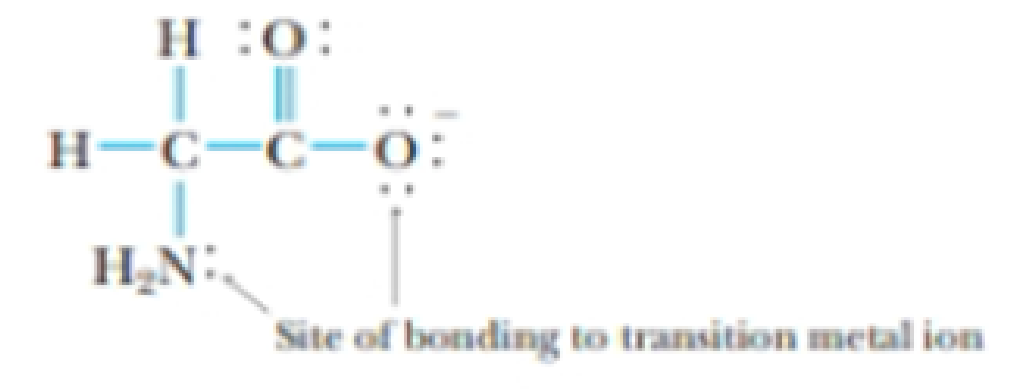Chapter 22, Problem 78GQ

Chapter
Section
Textbook Problem

The glycinate ion, H2NCH2CO2−, formed by deprotonation of the amino acid glycine, can function as a bidentate ligand, coordinating to a metal through the nitrogen of the amino group and one of the oxygen atoms.Glycinate ion, a bidentate ligandA copper complex of this ligand has the formula Cu(H2NCH2CO2)2(H2O)2. For this complex, determine the following:(a) the oxidation state of copper(b) the coordination number of copper(c) the number of unpaired electrons(d) whether the complex is diamagnetic or paramagnetic

(a)

Interpretation Introduction

Interpretation: The given questions to be answered.

Concept introduction:

Spectrochemical series:  A list of ligands ordered in the basis of ligand strength.

I-<Br-<Cl-<F-< OH-< H2O < NH3< en <CN-<CO

Paramagnetic property: The presence of unpaired electrons in the electronic configuration of the metal ion in coordination complex.

Diamagnetic property: The absence of unpaired electrons of the metal ion in coordination compound which do repel by the magnet.

Explanation

Cu(H2NCH2CO2)2(H2O)2Theoxidationstateofcopper:Chargeofligand(H2NCH2CO2)=-1

b.

Interpretation Introduction

Interpretation: The given questions to be answered.

Concept introduction:

Spectrochemical series:  A list of ligands ordered in the basis of ligand strength.

I-<Br-<Cl-<F-< OH-< H2O < NH3< en <CN-<CO

Paramagnetic property: The presence of unpaired electrons in the electronic configuration of the metal ion in coordination complex.

Diamagnetic property: The absence of unpaired electrons of the metal ion in coordination compound which do repel by the magnet.

c.

Interpretation Introduction

Interpretation: The given questions to be answered.

Concept introduction:

Spectrochemical series:  A list of ligands ordered in the basis of ligand strength.

I-<Br-<Cl-<F-< OH-< H2O < NH3< en <CN-<CO

Paramagnetic property: The presence of unpaired electrons in the electronic configuration of the metal ion in coordination complex.

Diamagnetic property: The absence of unpaired electrons of the metal ion in coordination compound which do repel by the magnet.

d.

Interpretation Introduction

Interpretation: The given questions to be answered.

Concept introduction:

Spectrochemical series:  A list of ligands ordered in the basis of ligand strength.

I-<Br-<Cl-<F-< OH-< H2O < NH3< en <CN-<CO

Paramagnetic property: The presence of unpaired electrons in the electronic configuration of the metal ion in coordination complex.

Diamagnetic property: The absence of unpaired electrons of the metal ion in coordination compound which do repel by the magnet.

Still sussing out bartleby?

Check out a sample textbook solution.

See a sample solution

The Solution to Your Study Problems

Bartleby provides explanations to thousands of textbook problems written by our experts, many with advanced degrees!

Get Started

Find more solutions based on key concepts